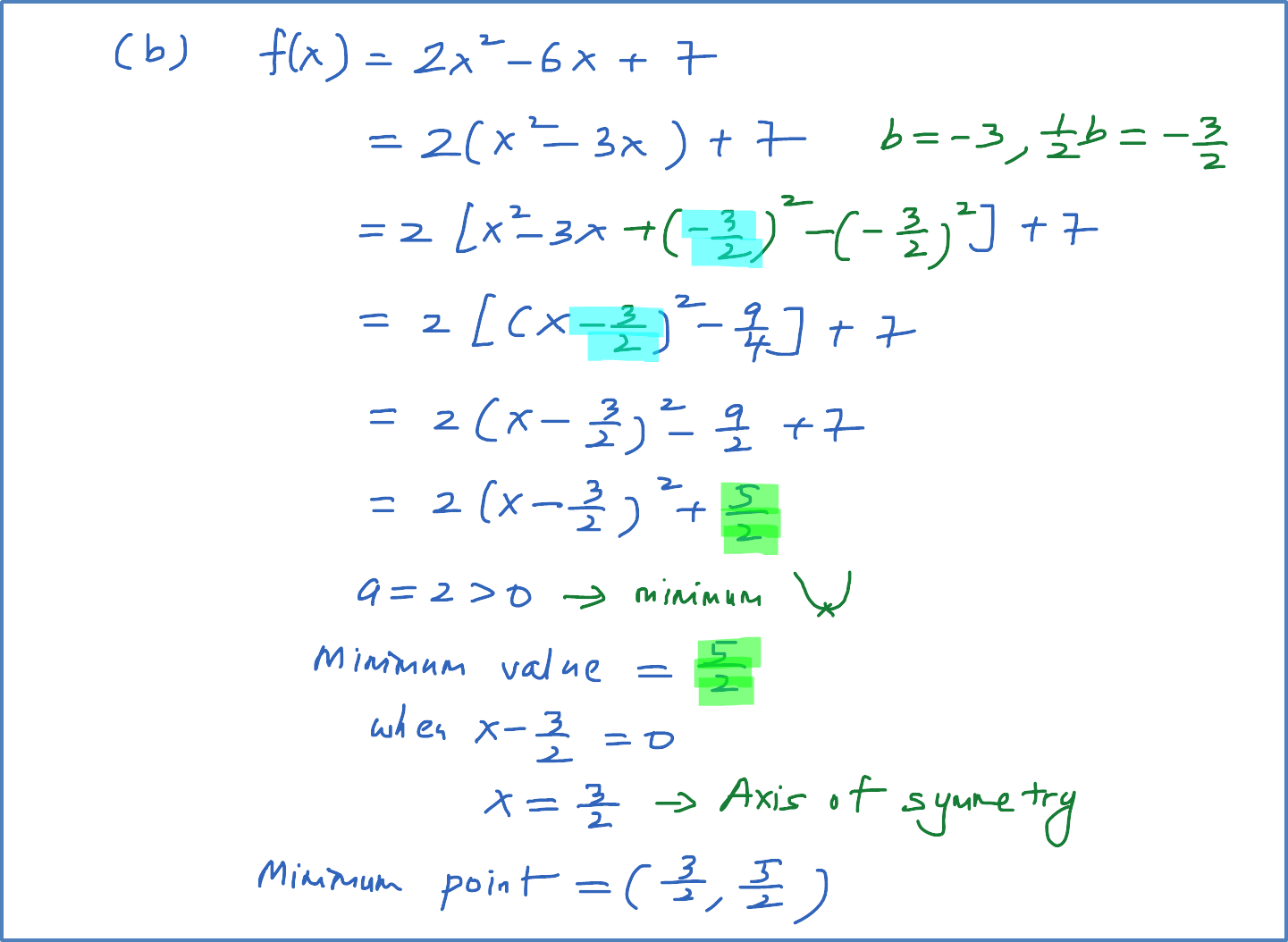# 2.4.1 Example 1 : Finding the Maximum and Minimum points of Quadratic Function using completing the square

Example 1
Find the maximum or minimum value of each of the following quadratic function by completing the squares.  In each case, state the value of x at which the function is maximum or minimum.  And also, state the maximum or minimum point and axis of symmetry for each case.

(a) $f\left(x\right)={x}^{2}+6x+7$
(b) $f\left(x\right)=2{x}^{2}-6x+7$
(c) $f\left(x\right)=5-2x-{x}^{2}$
(d) $f\left(x\right)=4+12x-3{x}^{2}$